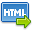Volume No. :   5

Issue No. :  1

Year :  2013

ISSN Print :  0975-4393

ISSN Online :  2349-2988Registration

Allready Registrered
Click to Login

## FeketeSzego ̈ functional for a subclass of starlike functions with respect to symmetric points

 Address: Harjinder Singh Department of Mathematics, Govt. Rajindra College, Bathinda (Punjab) *Corresponding Author
DOI No:

ABSTRACT:
Let?? be the class of functionsf(z)=z+?_(n=2)^8¦?a_n z^n ?analytic in the unit discE={z:|z|<1}. Let S(a) denote the class of functions in ?? satisfying the condition Re{((1-a)zf^' (z))/(f(z)-f(-z) )+?a(zf^' (z) )?^'/(f(z)-f(-z) )^' }> 0,(0=a=1)and z?E. We are interested in determining the upper bounds of the FeketeSzego ¨ functional |a_3-µa_2^2 | for functions of the class S(a). MATHEMATICS SUBJECT CLASSIFICATION:30C45
KEYWORDS:
Analytic functions, Carathe ́odory functions, Fekete Szego ̈ problem,Starlike functions with respect to symmetrical points.
Cite:
Harjinder Singh. FeketeSzego ̈ functional for a subclass of starlike functions with respect to symmetric points. Research J. Science and Tech 5(1): Jan.-Mar.2013 page 110-112.[View HTML][View PDF]

Visitor's No. :   147613